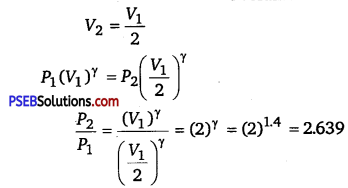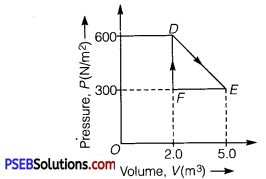# PSEB 11th Class Physics Solutions Chapter 12 Thermodynamics

Punjab State Board PSEB 11th Class Physics Book Solutions Chapter 12 Thermodynamics Textbook Exercise Questions and Answers.

## PSEB Solutions for Class 11 Physics Chapter 12 Thermodynamics

PSEB 11th Class Physics Guide Thermodynamics Textbook Questions and Answers

Question 1.
A geyser heats water flowing at the rate of 3.0 liters per minute from 27°C to 77°C. If the geyser operates on a gas burner, what is the rate of consumption of the fuel if its heat of combustion is 4.0 x 104 J/g?
Solution:
Water is flowing at a rate of 3.0 liter/min.
The geyser heats the water, raising the temperature from 27°C to 77°C.
Initial temperature, T1 = 27°C
Final temperature, T2 = 77°C
Rise in temperature, ΔT = T2 -T1
= 77-27 = 50°C

Heat of combustion = 4 x 104 J/g
Specific heat of water, C = 4.2 J g-1 °C-1
Mass of flowing water, m = 3.0 litre/min = 3000 g/min
Total heat used, ΔQ = mC ΔT
= 3000 x 4.2 x 50
= 6.3 x 105 J/min
∴ Rate of consumption = $$\frac{6.3 \times 10^{5}}{4 \times 10^{4}}$$ = 15.75 g/min

Question 2.
What amount of heat must be supplied to 2.0 x 10-2 kg of nitrogen (at room temperature) to raise its temperature by 45°C at constant pressure? (Molecular mass of N2 = 28; R = 8.3 J mol-1K-1).
Solution:
Mass of nitrogen, m = 2.0 x 10-2 kg = 20g
Rise in temperature, ΔT = 45°C
Molecular mass of N2,M =28
Universal gas constant, R = 8.3 J mol-1K-1
Number of moles, n = $$\frac{m}{M}$$
= $$\frac{2.0 \times 10^{-2} \times 10^{3}}{28}$$ = 0.714
Molar specific heat at constant pressure for nitrogen,
Cp = $$\frac{7}{2}$$ R = $$\frac{7}{2}$$ x 8.3
= 29.05J mol-1 K-1

The total amount of heat to be supplied is given by the relation
ΔQ = nCpΔT
= 0.714 x 29.05 x 45 = 933.38 J
Therefore, the amount of heat to be supplied is 933.38 JQuestion 3.
Explain why
(a) Two bodies at different temperatures T1 and T2 if brought in thermal contact do not necessarily settle to the mean temperature (T1 + T2) / 2.
(b) The coolant in a chemical or a nuclear plant (i.e., the liquid used to prevent the different parts of a plant from getting too hot) should have high specific heat.
(c) Air pressure in a car tyre increases during driving.
(d) The climate of a harbour town is more temperate than that of a town in a desert at the same latitude.
(a) When two bodies at different temperatures T1 and T2 are brought in thermal contact, heat flows from the body at the higher temperature to the body at the lower temperature till equilibrium is achieved, i.e., the temperatures of both the bodies become equal. The equilibrium temperature is equal to the mean temperature (T1 + T2) / 2 only when the thermal capacities of both the bodies are equal.

(b) The coolant in a chemical or nuclear plant should have a high specific heat. This is because higher the specific heat of the coolant, higher is its heat-absorbing capacity and vice versa. Hence, a liquid having a high specific heat is the best coolant to be used in a nuclear or chemical plant. This would prevent different parts of the plant from getting too hot.

(c) When a car is in motion, the air temperature inside the car increases because of the motion of the air molecules. According to Charles’ law, temperature is directly proportional to pressure. Hence, if the temperature inside a tyre increases, then the air pressure in it will also increase.

(d) A harbour town has a more temperate climate (i.e., without the extremes of heat or cold) than a town located in a desert at the same latitude. This is because the relative humidity in a harbour town is more than it is in a desert town.

Question 4.
A cylinder with a movable piston contains 3 moles of hydrogen at standard temperature and pressure. The walls of the cylinder are made of a heat insulator, and the piston is insulated by having a pile of sand on it. By what factor does the pressure of the gas increase if the gas is compressed to half its original volume?
Solution:
The cylinder is completely insulated from its surroundings. As a result, no heat is exchanged between the system (cylinder) and its surroundings. Thus, the process is adiabatic.
Initial pressure inside the cylinder = P1
Final pressure inside the cylinder = P2
Initial volume inside the cylinder = V1
Final volume inside the cylinder = V2
Ratio of specific heats, γ = 1.4 For an adiabatic process,
we have
P1V1γ = P2V2γ
The final volume is compressed to half of its initial volume.Hence, the pressure increases by a factor of 2.639.

Question 5.
In changing the state of a gas adiabatically from an equilibrium state A to another equilibrium state B, an amount of work equal to 22.3 J is done on the system. If the gas is taken from state A to B via a process in which the net heat absorbed by the system is 9.35 cal, how much is the net work done by the system in the latter case? (Take 1 cal = 4.19 J)
Solution:
The work done (W) on the system while the gas changes from state A to state Bis 22.3 J.
This is an adiabatic process. Hence, change in heat is zero.
ΔQ = 0
ΔW = -22.3 J (since the work is done on the system)
From the first law of thermodynamics, we have:
ΔQ = ΔU + ΔW

where, ΔU = Change in the internal energy of the gas .
ΔU = ΔQ – ΔW = -(-22.3 J)
ΔU = +22.3 J
When the gas goes from state A to state B via a process, the net heat absorbed by the system is
ΔQ =9.35cal = 9.35 x 4.19 = 39.1765J
Heat absorbed, ΔQ = ΔU + ΔW
ΔW = ΔQ – ΔU = 39.1765 – 22.3 – = 16.8765J
Therefore, 16.88 J of work is done by the system.Question 6.
Two cylinders A and B of equal capacity are connected to each other via a stopcock.
A contains a gas at standard temperature and pressure.
B is completely evacuated. The entire system is thermally insulated.
The stopcock is suddenly opened. Answer the following:
(a) What is the final pressure of the gas in A and B?
(b) What is the change in internal energy of the gas?
(c) What is the change in the temperature of the gas?
(d) Do the intermediate states of the system (before settling to the final equilibrium state) lie on its P -V – T surface?
Solution:
(a) 0.5 atm
The volume available to the gas is doused as soon as the stopcock between cylinders A and B is opened? Since volume is inversely proportional to pressure, the pressure will decrease to one-half of the original value. Since the initial pressure of the gas is 1 atm, the pressure in each cylinder will be 0.5atm.

(b) Zero
The internal energy of the gas can change only when work is done by or on the gas. Since in this case no work is done by or on the gas, the internal energy of the gas will not change.

(c) Zero
Since no work is being done by the gas during the expansion of the gas, the temperature of the gas will not change at all.

(d) No
The given process is a case of free expansion. It is rapid and cannot be controlled. The intermediate states do not satisfy the gas equation and since they are in non-equilibrium states, they do not lie on the P-V-T surface of the system.

Question 7.
A steam engine delivers 5.4 x 108 J of work per minute and services 3.6 x 109 J of heat per minute from its boiler. What is the efficiency of the engine? How much heat is wasted per minute?
Solution:
Work done by the steam engine per minute, W = 5.4 x 108 J
Heat supplied from the boiler, H = 3.6 x 109 J
Efficiency of the engine = $$\frac{\text { Output energy }}{\text { Input energy }}$$
∴ η = $$\frac{W}{H}=\frac{5.4 \times 10^{8}}{3.6 \times 10^{9}}$$ = 0.15
Hence, the percentage efficiency of the engine is 15%.
Amount of heat wasted= 3.6 x 109 – 5.4 x 108
= 30.6 x 108 = 3.06 x 109 J
Therefore, the amount of heat wasted per minute is 3.06 x 109J.

Question 8.
An electric heater supplies heat to a system at a rate of 100 W. If system performs work at a rate of 75 joules per second. At what rate is the internal energy increasing?
Solution:
Heat is supplied to the system at a rate of 100 W.
∴ Heat supplied, ΔQ = 100 J/s
The system performs at a rate of 75 J/s.
∴ Work done, ΔW = 75 J/s

From the first law of thermodynamics, we have
ΔQ = ΔU + ΔW
where ΔU = Rate of change in internal energy
ΔU = ΔQ – ΔW = 100 – 75 = 25 J/s = 25W
Therefore, the internal energy of the given electric heater increases at a rate of 25 W.Question 9.
A thermodynamic system is taken from an original state to an intermediate state by the linear process shown in figure given below.Its volume is then reduced to the original value from E to F by an isobaric process. Calculate the total work done by the gas from D to E to F.
Solution:
Total work done by the gas from D to E to F = Area of ΔDEF
Area of ΔDEF = $$\frac{1}{2}$$ DF x EF
where, DF = Change in pressure
=600 N/m2
= 300N/m2 = 300N/m2
FE = Change in volume
5.0 m3 – 2.0 m3 = 3.0m3
Area of ADEF = $$\frac{1}{2}$$ x 300 x 3 = 450 J
Therefore, the total work done by the gas from D to E to F is 450 J.

Question 10.
A refrigerator is to maintain eatables kept inside at 9°C. If the room temperature is 36°C, Calculate the coefficient of performance.
Solution:
Temperature inside the refrigerator, T1 = 9°C = 273 + 9 = 282 K
Room temperature, T2 = 36°C = 273+36
Coefficient of performance = $$\frac{T_{1}}{T_{2}-T_{1}}$$
= $$\frac{282}{309-282}=\frac{282}{27}$$
309-282 = 10.44
Therefore, the coefficient of performance of the given refrigerator is 10.44.